MCAT Physical : Thermochemistry and Energetics

Example Questions

← Previous 1 3 4 5 6 7 8

Example Question #1 : Thermochemistry And Energetics

Adenosine-triphosphate is formed by the following endothermic reaction.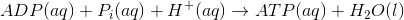If body temperature decreases, ADP production __________.

remains constant

decreases

increases

is unpredictable

increases

Explanation:

Since heat can be considered a reactant (endothermic), if heat is decreased, ADP is increased according to Le Chatelier’s Principle. If a reactant is removed, the equilibrium will shift toward the reactants. In this reaction, ADP and heat are both reactants.

Example Question #1 : Thermochemistry And Energetics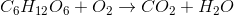Which of the following is true, assuming the reaction goes to completion?

I. There is an increase in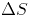, entropy

II.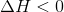III. At equilibrium,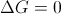IV. Oxygen is the reducing agent

II and III

II only

I and III

I, II, and III

I and IV

I, II, and III

Explanation:

This question ties together the reaction for oxidation of glucose with several thermodynamic principles.

The first option, stating that there is an increase of entropy, is true. When balanced, we see that there is an increase in total number of molecules when the reaction completes; thus, there will be an increase in entropy.

The second option states that enthalpy, or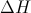, is less than than zero. This is also true, as it implies that this is an exothermic (or heat generating) reaction. The oxidation of glucose is how our bodies generate heat and keep homeostasis.

The third option, stating that Gibb's free energy is zero at equilibrium, is also true. Any equation will haveat equilibrium.

The last option, stating that oxygen is the reducing agent, is false. Oxygen is actually the oxidizing agent and is itself reduced.

Example Question #1 : Energetics Of Biological Reactions And Atp

ATP stores energy through its ability to lose a phosphate group to form ADP in the following reaction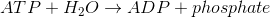At body temperature, the thermodynamic values for ATP hydrolysis are as follow.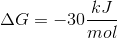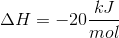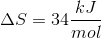Glycolysis is an energy-liberating process (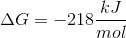,) that is coupled with the conversion of two ADP molecules into two ATP molecules according to the following reaction.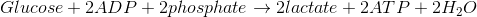Glycolysis results in which of the following?

An increase in entropy

A conservation of entropy

An increase in enthalpy

A decrease in entropy

An increase in entropy

Explanation:

Since glycolysis is an exothermic reaction (ΔH is negative), enthalpy does not increase. As for entropy, the reaction begins with a large molecule and ends with smaller molecules, therefore entropy increases.

Example Question #4 : Thermochemistry And Energetics

ATP stores energy through its ability to lose a phosphate group to form ADP in the following reactionAt body temperature, the thermodynamic values for ATP hydrolysis are as follow.
ΔG = –30kJ/mol
ΔH = –20kJ/mol
ΔS = 34kJ/mol

Glycolysis is an energy-liberating process (ΔG = –218kJ/mol, ΔH = –20 kJ/mol) that is coupled with the conversion of two ADP molecules into two ATP molecules according to the following reaction.Glycolysis is __________.

exergonic and exothermic

exergonic and endothermic

endergonic and endothermic

endergonic and exothermic

exergonic and exothermic

Explanation:

ΔG and ΔH are both negative for glycolysis, meaning that the reaction is exergonic and exothermic.

Example Question #5 : Thermochemistry And Energetics

ATP stores energy through its ability to lose a phosphate group to form ADP in the following reactionAt body temperature, the thermodynamic values for ATP hydrolysis are as follow.
ΔG = –30kJ/mol
ΔH = –20kJ/mol
ΔS = 34kJ/mol

Glycolysis is an energy-liberating process (ΔG = –218kJ/mol, ΔH = –20 kJ/mol) that is coupled with the conversion of two ADP molecules into two ATP molecules according to the following reaction.The formation of peptide bonds requires 17kJ/mol of energy. It also requires the energy of three ATP molecules. How many ATP molecules are required to synthesize a protein containing 550 peptide bonds?

550

1,962

1,650

312

1,962

Explanation:

Since we know that three ATP molecules per bond are required, 1,650 ATP molecules are needed. However, we also know that 17kJ/mol of additional energy is required for each bond. This energy must come from ATP molecules. The only choice that is greater than 1,650 is 1,962.

Example Question #6 : Thermochemistry And Energetics

ATP stores energy through its ability to lose a phosphate group to form ADP in the following reactionAt body temperature, the thermodynamic values for ATP hydrolysis are as follow.
ΔG = –30kJ/mol
ΔH = –20kJ/mol
ΔS = 34kJ/mol

Glycolysis is an energy-liberating process (ΔG = –218kJ/mol, ΔH = –20 kJ/mol) that is coupled with the conversion of two ADP molecules into two ATP molecules according to the following reaction.How much heat, in joules, is released when 4 * 1019 molecules of ATP are converted to ADP via ATP hydrolysis?

45J

13,000J

1.3 * 10-3J

1.3J

1.3J

Explanation:

To solve this problem, first convert molecules to moles with Avogadro’s number, then convert moles to kJ using the ΔH of ATP hydrolysis. Lastly convert kJ to J.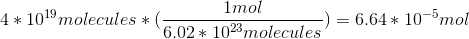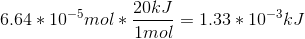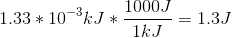Example Question #1 : Gibbs Free Energy

A scientist prepares an experiment to demonstrate the second law of thermodynamics for a chemistry class. In order to conduct the experiment, the scientist brings the class outside in January and gathers a cup of water and a portable stove.

The temperature outside is –10 degrees Celsius. The scientist asks the students to consider the following when answering his questions:

Gibbs Free Energy Formula:

ΔG = ΔH – TΔS

Liquid-Solid Water Phase Change Reaction:

H2O(l) ⇌ H2O(s) + X

The scientist prepares two scenarios.

Scenario 1:

The scientist buries the cup of water outside in the snow, returns to the classroom with his class for one hour, and the class then checks on the cup. They find that the water has frozen in the cup.

Scenario 2:

The scientist then places the frozen cup of water on the stove and starts the gas. The class finds that the water melts quickly.

After the water melts, the scientist asks the students to consider two hypothetical scenarios as a thought experiment.

Scenario 3:

Once the liquid water at the end of scenario 2 melts completely, the scientist turns off the gas and monitors what happens to the water. Despite being in the cold air, the water never freezes.

Scenario 4:

The scientist takes the frozen water from the end of scenario 1, puts it on the active stove, and the water remains frozen.

In scenario 1, the reaction of water freezing has which of the following quantities for the Gibbs Free Energy Formula?

Enthalpy: Negative; Entropy: Zero

Enthalpy: Negative; Entropy: Negative

Enthalpy: Negative; Entropy: Positive

Enthalpy: Positive; Entropy: Negative

Enthalpy: Positive; Entropy:  Positive

Enthalpy: Negative; Entropy: Negative

Explanation:

As water freezes, heat is released. The H value in the equation must therefore be negative.  Also as water freezes, there is a local decline in the amount of disorder. The S, or entropy, term in the Gibbs equation refers to entropy of the system. Thus, this term must be negative as well.

Example Question #2 : Gibbs Free Energy

Diffusion can be defined as the net transfer of molecules down a gradient of differing concentrations. This is a passive and spontaneous process and relies on the random movement of molecules and Brownian motion. Diffusion is an important biological process, especially in the respiratory system where oxygen diffuses from alveoli, the basic unit of lung mechanics, to red blood cells in the capillaries.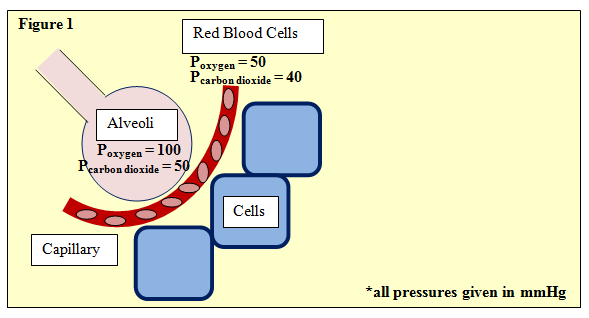Figure 1 depicts this process, showing an alveoli separated from neighboring cells by a capillary with red blood cells. The partial pressures of oxygen and carbon dioxide are given. One such equation used in determining gas exchange is Fick's law, given by:

ΔV = (Area/Thickness) · Dgas · (P1 – P2)

Where ΔV is flow rate and area and thickness refer to the permeable membrane through which the gas passes, in this case, the wall of the avlveoli. P1 and P2 refer to the partial pressures upstream and downstream, respectively. Further, Dgas­, the diffusion constant of the gas, is defined as:

Dgas = Solubility / (Molecular Weight)^(1/2)

In any diffusion process, what is ΔG, Gibbs energy?

ΔG = 0

ΔG ≤ 0

Cannot be determined

ΔG ≥ 0

ΔG ≤ 0

Explanation:

The key here is that diffusion, as mentioned in the passage, is a passive or spontaneous process. That is, it occurs without any energy expenditure, and therefore for any diffusion process, ΔG must be less than 0. In contrast, other transport mechanisms, such as active transport, require ATP as an energy source, and therefore ΔG ≥ 0.

Example Question #3 : Gibbs Free Energy

A scientist is studying a reaction, and places the reactants in a beaker at room temperature. The reaction progresses, and she analyzes the products via NMR. Based on the NMR readout, she determines the reaction proceeds as follows: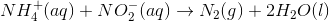In an attempt to better understand the reaction process, she varies the concentrations of the reactants and studies how the rate of the reaction changes. The table below shows the reaction concentrations as she makes modifications in three experimental trials.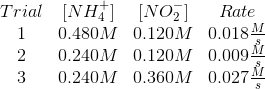After beginning the reaction described in the passage, a scientist waits as it comes to equilibrium at standard conditions. He calculates the equilibrium constant to be much greater than 1. Which of the following must be true?

The standard free energy change of the reaction is greater than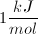The standard free energy change of the reaction is positive

The standard free energy change of the reaction is between zero andThe standard free energy change of the reaction is negative

The standard free energy change of the reaction is zero

The standard free energy change of the reaction is negative

Explanation:

If the equilibrium constant of a reaction is much greater than 1, its equilibrium is characterized by having a relative abundance of products to reactants.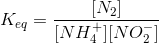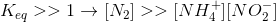This ratio implies that the reaction is spontaneous, and can be formally stated in terms of Gibbs free energy.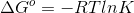Example Question #1 : Thermochemistry And Energetics

Which of the following conditions will result in a spontaneous reaction?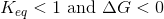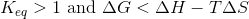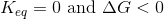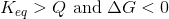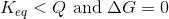Explanation:

This question tests two distinct, but related, principles: equilibrium constant and Gibbs free energy.

Equilibrium constant is given by the following equation, only when the concentrations represent equilibrium quantities of the compounds involved: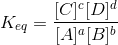In contrast, the reaction quotient is determined by this same equation when the compounds are not in equilibrium. What this means is that a reaction with a reaction quotient greater than the equilibrium constant at a given moment has an excess of products, compared to equilibrium. In contrast, a reaction with a reaction quotient less than the equilibrium constant has a relative abundance of reactions. In this later scenario, the reaction will proceed toward the products in order to achieve equilibrium.

An equilibrium constant greater than one indicates that there are more products than reactants at equilibrium, thus favoring the forward reaction and indicating spontaneity.

In summary, the equilibrium constant must be greater than the reaction quotient or greater than one in order for the reaction to be considered spontaneous.

Evaluating Gibbs free energy is much simpler: a negative value for Gibbs free energy indicates a spontaneous reaction. The equation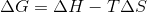is always true, and the inequality listed in one of the answer choices cannot exist.

← Previous 1 3 4 5 6 7 8